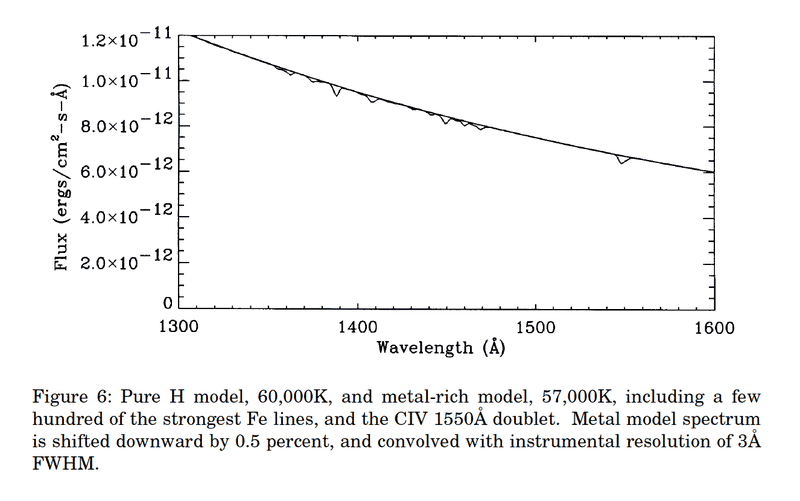# Computing a value of radius for a typical white dwarf

• I
I try to get a correct result for the radius of a standard white dwark (roughly 10000 km).

I just want the order of magnitude , i.e with the common values of a solar mass into earth radius sphere.

From http://www.astro.umontreal.ca/~bergeron/CoolingModels/Synthetic_Calibration.pdf page 1223, I took the following (typical ?) values to compute this radius :

##\Phi_{\text{bolometric}}=10^{-9}\,\text{erg}.\text{cm}^{-2}.\text{s}^{-1}##

##T_{\text{surface}}=10000 K##, ##\text{D}=140## parsec,

and by using the following formula :

##R=\sqrt{\dfrac{ \Phi_{\text{bolometric}} D^{2}}{\sigma T^{4}}}##

Then I calculate the radius with all these values in (SI) units ##(1\,erg.cm^{-2} = 10^{-7} 10^{4}\,J.m^{-2}=10^{-3}\,J.m^{-2}##

$$Radius = \sqrt{\dfrac{(10^{-9}*10^{-7}*10^4*(140*3.26*3600*24*365*3*10^8)^2)}{(5.67*10^{-8}*(10000^4)}}$$

and I get ##Radius = 1.8134\,10^8 \,\text{meters} = 1.8134\,10^{5} \,\text{kilometers}##

That's too high as result, I expect rather a scale ##10^{3}## km < Radius < ##10^{4}## km.

If someone could tell me where is my error to get a standard value of radius for a white dwarf ?

UPDATE :

I get expected results with a flux equal to : ##\Phi_{\text{bolometric}}=10^{-12}## erg.s^-1.cm^-2

such that :

= 5.7343 10^3 km

Anyone could confirm me the typical value of a bolometric flux (apparent brightness) equal to ##10^{-12}##erg.s^-1.cm^-2 for a white dwarf distant from 140 pc ?

I have difficulties to do the link between the monochromatic spectral flux (exprimed in erg.cm^-2.s^-1.Angstrom^-1) and the total flux ( in erg.cm^-2.s^-1, i.e the monochromatic spectral flux integrated on all wavelength), like for example in this figure :But from this figure, I can only get the total flux between 1300 and 1600 Angstrom, not the total flux over all wavelengths (##\Phi_{\text{bolometric}}(1300<\lambda<1600)\,\approx\,8*10^{-12}*300\,\approx\,2.4*10^{-9}\, erg.cm^-2.s^-1##)

Any help or suggestion is welcome, regards

#### Attachments

stefan r
That is a lot to plug into a calculator.

Parsec ≈ 31 petameters. 3.1 x 1016

square parsec is 931 square petameters, or 9.6 x 1032m

10-12 x 1402 x 9.6 x 1032 = 1.88 x 1025

1.88 x 1025/(5.67 x 108)= 3.3 x 1016

√(3.3 x 1012) = 181659021 ≈ 1.8 x 108

That looks O.K.

Should your equation have a 4π next to σ?

The table on page 1223 of your link has values for Vega magnitude. You should be calculating Vega's radius.

That is a lot to plug into a calculator.

Parsec ≈ 31 petameters. 3.1 x 1016

square parsec is 931 square petameters, or 9.6 x 1032m

10-12 x 1402 x 9.6 x 1032 = 1.88 x 1025

1.88 x 1025/(5.67 x 108)= 3.3 x 1016

√(3.3 x 1012) = 181659021 ≈ 1.8 x 108

That looks O.K.

Should your equation have a 4π next to σ?

The table on page 1223 of your link has values for Vega magnitude. You should be calculating Vega's radius.

@stefan r

It seems that you have not used the conversion between erg and SI units since ##\sigma = 5.67 10^{-8} \,W.m^{-2}##, haven't you ?

Regards

stefan r
@stefan r

It seems that you have not used the conversion between erg and SI units since ##\sigma = 5.67 10^{-8} \,W.m^{-2}##, haven't you ?

Regards
I used the same numbers you had. 5.67 x 10-8 x (104)4 = 5.67 x 108

σ has units W m-2s-1T-4 but that works fine in the equation in your first post.

@stefan r

Sorry, I must precise that in the calculation :

I apply the factor "##10^{-7}*10^{4}##" since the bolometric flux is expressed as : ##\Phi_{\text{bolometric}}=10^{-12}\,\text{erg.cm^-2.s^-1} = 10^{-12}\,10^{-7}\,\text{J.cm^-2.s^-1} = 10^{-12}\,10^{-7}\,10^{4}\,\text{W.m^-2}=10^{-15}\,\text{W.m^-2}##

I hope you understand